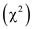# Quiz 11: Population Growth

Geology/Geography/Oceanography/Atmospheric Sciences

The populations may grow geometrically or exponentially in nature for a specific period of time. A geometric model of population growth is shown by annual species (those which complete their life cycle in a year). They usually produce a single generation in a year. Hence, the generations do not overlap. The organisms showing the geometric model of population growth include the annual plant species and most of the species of insects. An exponential model of population growth is shown by species that show continuous or nonpulsed reproduction. Their populations are made up of overlapping generations. The organisms showing exponential model of population growth include humans, bacteria, and species of birds, fish, and trees. The populations of species capable of growing exponentially may do so only under certain conditions for a specific period of time. These conditions are as follows: • Favorable environmental conditions with abundant nutrients and space availability • Low population density • Introduction in a new territory • Favorable but transient variations in the climate • Conservation strategies The population will not grow exponentially under following circumstances: • Unfavorable environment with scarcity of food and water • High population density • Competition for common resources, high rate of predation, prevalence of disease and parasitism. • Human intervention in the form of hunting, and habitat destruction.

A hypothesis testing may involve comparison of the observed frequencies and the expected or hypothetical frequencies. The testing of hypothesis involving relationship between the hypothetical and observed frequencies can be done by a method called chi-squaretest or "goodness of fit" test. The test can be used to study whether the observed frequencies match with the expected frequencies. The test was used to study the relationship between the observed frequencies and the expected frequencies of alternative male phenotypes of a lizard species. The analysis was found to show significant differences between the male phenotypes at the significance level 0.05. If the significance level of P=0.01 is chosen at degrees of freedom equal to 2, the critical value of chi-squarefrom the statistical table would be 9.210. Since the calculated value of chi-squarethat is 15.17 is greater than 9.210, the hypothesis indicating equal frequencies of all the male phenotypes of the lizard species is rejected. So, the result of the analysis by "goodness of fit" remains significant at both the significance levels of P = 0.05 and P = 0.01.

The growth of a population can be assessed by studying the parameters of the population such as the net reproductive rate, the generation time, the geometric rate of increase, and per capita rate of increase. Despite many efforts of protection, the population size of the right whale has remained small. This shows a slower growth rate when compared to the other species of the whale. If the external factors such as the environmental factors are excluded from the study, the slow growth rate of the right whale population can be explained by estimation of the population parameters. The right whale populations are found to reproduce once in a span of 4 to 7 years. This indicates a pulsed reproduction and geometric pattern of growth. The other information needed for explaining the slow growth of the right whale population can be due to their birth rate and the death rate of the population. The estimation of various population parameters needs information about the survivorship and number of individuals of each age class. This information can help to determine the net reproductive rate, the generation time, the geometric rate of increase, and the per capita rate of increase. The values of population parameter such as per capita rate of increase can explain the population growth. A positive value of per capita rate of increase indicates a growing population whereas a negative value indicates a declining population.